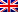# Seminario di Fisica Matematica

Abstract: We study a lattice gas subject to Kawasaki dynamics at inverse temperature $\beta>0$ in a large finite box $\Lambda_\beta \subset \mathbb{Z}^2$. Each pair of neighbouring particles has a negative binding energy $-U<0$, while each particle has a positive activation energy $\Delta>0$. The initial configuration is drawn from the grand-canonical ensemble restricted to the set of configurations where all the droplets are subcritical. Our goal is to describe, in the metastable regime $\Delta \in (U,2U)$ and in the limit as $\beta\to\infty$, how subcritical droplets form and dissolve when the volume is moderately large, namely $|\Lambda_\beta| = e^{\theta\beta}$ with $\Delta < \theta < 2\Delta-U$. We will see that the evolution of the gas consists of droplets wandering around on multiple space-time scales in a way that can be captured by a coarse-grained Markov chain on a space of droplets. This is a joint work with Alexandre Gaudillière, Frank den Hollander, Francesca Romana Nardi, Enzo Olivieri and Elisabetta Scoppola.
This post is also available in: Link identifier #identifier__96746-5Eng